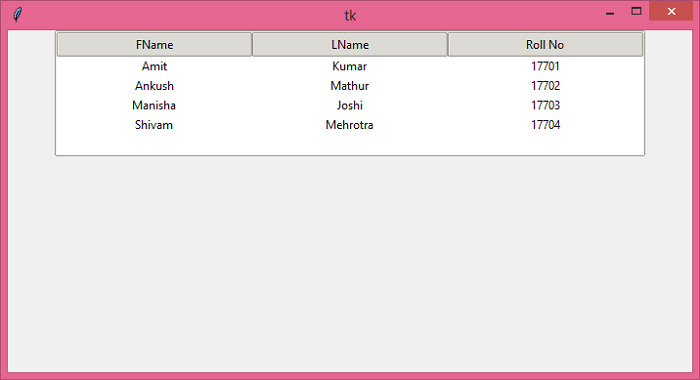# What is the best way to show data in a table in Tkinter?

Generally, we represent data in the form of tables. A table contains a set of rows and columns. Data gets stored sequentially in the form of rows and columns in a table.

Let us suppose that we are building a Tkinter application such that we have to store the Student’s data somewhere in a table. The table structure contains 3 columns to store the First Name, Last Name, and Roll Number of students. To display this type of information, Tkinter provides a Notebook widget where we can store our data in the form of a Table.

## Example

# Import the required libraries
from tkinter import *
from tkinter import ttk

# Create an instance of tkinter frame
win = Tk()

# Set the size of the tkinter window
win.geometry("700x350")

# Create an object of Style widget
style = ttk.Style()
style.theme_use('clam')

tree = ttk.Treeview(win, column=("FName", "LName", "Roll No"), show='headings', height=5)
tree.column("# 1", anchor=CENTER)
tree.column("# 2", anchor=CENTER)
tree.column("# 3", anchor=CENTER)

# Insert the data in Treeview widget
tree.insert('', 'end', text="1", values=('Amit', 'Kumar', '17701'))
tree.insert('', 'end', text="1", values=('Ankush', 'Mathur', '17702'))
tree.insert('', 'end', text="1", values=('Manisha', 'Joshi', '17703'))
tree.insert('', 'end', text="1", values=('Shivam', 'Mehrotra', '17704'))

tree.pack()

win.mainloop()

## Output

If we run the above code, it will display a window that contains a table with some student data in it.